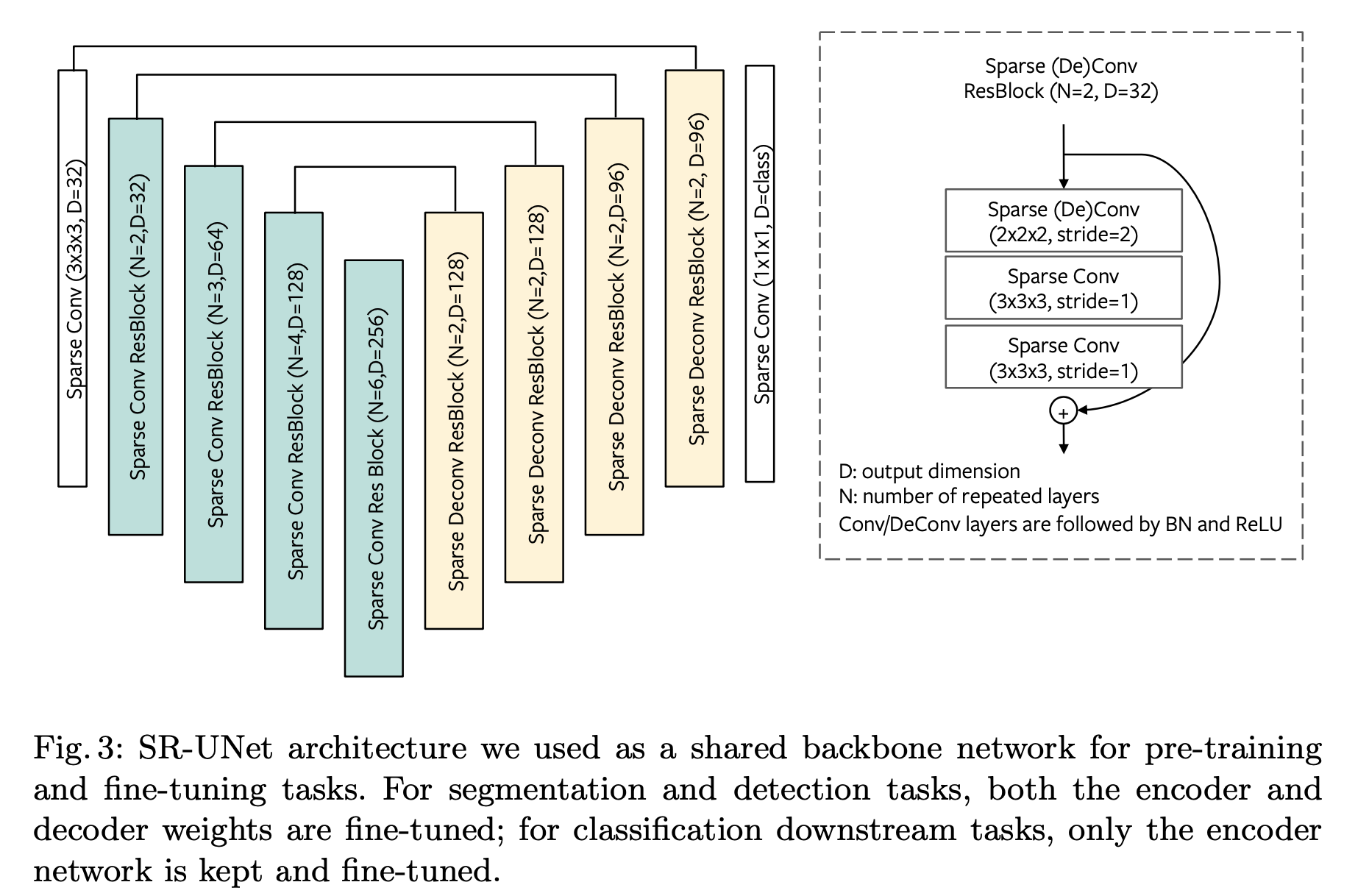# 3D Datasets

## Data Representations

1. Multiview images: multiple 2D images of the same object from different angles.
2. Depth map
3. Voxel: volumetric occupancy
4. Point cloud
5. Polygon mesh
6. Functions as implicit representation

## ScanNet Dataset

• Scene: IndoorScanNet is a richly-annotated RGB-D video dataset. It has camera poses information, surface reconstruction, sementic segmentation labels.

### Data Formats

#### *.ply Reconstructed surface mesh file

This is binary PLY format mesh with +Z axis in upright orientation.

#### *.sens RGB-D sensor stream

This is binary format with per-frame color, depth, camera pose and other data.

# Tools

## Minkowski Engine

The Minkowski Engine is an auto-differentiation library for sparse tensors. It supports all standard network layers such as convolution, pooling, unpooling, and broadcasting operations for sparse tensors.

3D voxel feature maps are often sparse. Minkowski Engine generalizes the definition of 3D sparse convolution and provide implementation support for it. The sparse convolution is defined as:

This definition does not limit the shape of the kernel.

• $\mathbf{u}$ is the current convolution center.
• $\mathcal{N}^D(\mathbf{u}, \mathcal{C}^{in})$ is a set of offsets that define the shape of a kernel, centered at $\mathbf{u}$.
• $\mathcal{C}^{in}$, $\mathcal{C}^{out}$ are predefined input and output coordinates of sparse tensors.

Minkowski Engine uses COO format (Coordinate list) to store sparse tensors, meaning that, it stores a list of non-zero coordinates and corresponding features:

### Concepts

Coordinate Manager
Because the feature map is sparse, we need to dynamically find neighbors between non-zero elements. Minkowski Engine has a mechanism called coordinate manager that caches and reuses calcualted neighbor coordinates. When a MinkowskiEngine.SparseTensor is initialized, a coordinate manager is also created. The coordinate manager can be accessed with MinkowskiEngine.SparseTensor.coords_man, and it can be shared with a new sparse tensor by providing it as an argument during initialization.

Channel
The channel number in 3D context is the length of the feature vector.

# Paper Digests

## PointContrast: Unsupervised Pre-Training for 3D Point Cloud Understanding

• Authors: Saining Xie, Jiatao Gu, Demin Guo, Charles R. Qi, Leonidas Guibas, Or Litany
• Venue: ECCV 2020
• Institution: Facebook AI & Stanford

### Motivation

To design a full pipeline for 3D unsupervised pretraining: view generation methods, downstream task compatible backbone, contrastive loss design. Previous work (pretraining on ShapeNet) doesn’t work because of two reasons: source & target data domain gap and lack of point-level representation.

### Methodology

PointContrast Pipeline: contrasting at point-level

1. Given point cloud $\mathbf{x}$, generate two views $\mathbf{x^1}$ and $\mathbf{x^2}$ that are aligned in the same world coordinates.
2. Compute the correspndence mapping $M$ between these two views. If $(i,j)\in M$ then points $x_i^1$ and $x_j^2$ are a matched pair.
3. Randomly sample two geometric transformations $\mathbf{T_1}$, $\mathbf{T_2}$ (translation, rotation, scaling), and apply each to the paired points.
4. Compute output point feature $\mathbf{f^1}=NN(\mathbf{T_1}(\mathbf{x^1}))$ and $\mathbf{f^2}=NN(\mathbf{T_1}(\mathbf{x^2}))$.
5. Backprop to update NN with point-wise contrastive loss.

Contrastive Loss Design
The intuition of contrastive loss is to seperate negative pairs and pull together positive pairs as much as possible.

Hardest-Contrastive Loss
• $\mathbf{f_i}$, $\mathbf{f_j}$ are the features of matched points.
• $d()$: Euclidean distance.
• $m_p$ and $m_n$ are positive/negative margins, they are hyperparameters.
• $\mathcal{N}$ is a randomly sampled set of non-matched (negative) points.
hardest sample means the closest point in the $\mathcal{L2}$ normalized feature space. Because the closest negative point in the feature space is the hardest to separate.
PointInfoNCE Loss

PointInfoNCE is derived from InfoNCE. InfoNCE treat contrastive learning as classification problem, thus implemented with cross-entropy loss.

• $\tau$, annealing temperature, $\tau \rightarrow \infty$ the output distribution goes “flat”, i.e. positive pair become less distinguishable. $\tau \rightarrow 0$ the output distribution goes sharp, i.e. positive pair becomes more distinguishable.

Backbone Design### Conclusions

#### Why Pretraining with ShapeNet dataset is not useful?

• Domain gap between source and target dataset: alignment, scene context …
• Point-level representation matters: local geometric feature is critical for 3D tasks

#### The advantages of fully-convolutional design

• Don’t have to crop objects out from scene context as done in previous works.
• Enables “point-level metric learning”.

What is “view” and how is it generated?”
View is partial point cloud seen from different viewpoints. We can generate two views by cropping them from a complete point cloud. “Aligning two views in the same world coordinates” means to register them, i.e., find the common matched points.

How do we know the mapping between output features?
The SRUNet’s output point cloud has the exact same coordinates with its input (just like segmentation). So we can use the input points’ mapping to map features in the output sparse tensors.

# Resources

## Paper Lists

3D-PointCloud GitHub Repo

3D related: View things from another dimension - Posted by Tianchen

# TODO

• My website’s syntax highlight theme sucks, I need to fix it